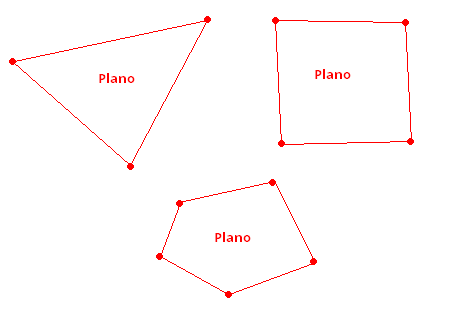# Create a plane from points

Hi Guys,

Please, I need help: I have n points (x, y, z), where n> 2

From these points, i need to build a plane. Any idea where I can start? This is possible?Thank you

I’m assuming you are talking about building a mesh in the shape defined by the vertices. If your polygon is axes aligned, and if it is convex, then it is fairly easy to do. If it is not axes aligned, then I’d likely rotate it to match an the axes, build the mesh, then rotate the vertices again. If it is not convex, then it becomes a much harder problem and you’ll likely have to dig around in some CompSci posts for the algorithm.

I would approach the problem by finding a center point and then defining the triangles in terms of this center point:

[16034-polytoplane.png*_|16034]

Here is a bit of source that will build polygons. Attach the script to an empty game object, define your polygon shape in the ‘poly’ array in the Inspector, and attach a material to display to the ‘mat’ parameter. The polygon is built on the XY plane and any ‘z’ components in the ‘poly’ Vector3s are ignored.

``````using UnityEngine;
using System.Collections;

public class PlaneFromPoly : MonoBehaviour {

public Material mat;
public Vector3[] poly;  // Initialized in the inspector

void Start () {
if (poly == null || poly.Length < 3) {
Debug.Log ("Define 2D polygon in 'poly' in the the Inspector");
return;
}

Mesh mesh = new Mesh();
mf.mesh = mesh;

rend.material = mat;

Vector3 center = FindCenter ();

Vector3[] vertices = new Vector3[poly.Length+1];
vertices = Vector3.zero;

for (int i = 0; i < poly.Length; i++) {
poly*.z = 0.0f;*
``````

_ vertices[i+1] = poly - center;_
* }*

* mesh.vertices = vertices;*

_ int triangles = new int[poly.Length*3];_

* for (int i = 0; i < poly.Length-1; i++) {*
_ triangles[i*3] = i+2;
triangles[i*3+1] = 0;
triangles[i*3+2] = i + 1;_

* }*

_ triangles[(poly.Length-1)*3] = 1;
triangles[(poly.Length-1)*3+1] = 0;
triangles[(poly.Length-1)*3+2] = poly.Length;_

* mesh.triangles = triangles = triangles;*
* mesh.uv = BuildUVs(vertices);*

* mesh.RecalculateBounds();*
* mesh.RecalculateNormals();*

* }*

* Vector3 FindCenter() {*
* Vector3 center = Vector3.zero;*
* foreach (Vector3 v3 in poly) {*
* center += v3; *
* }*
* return center / poly.Length;*
* }*

* Vector2[] BuildUVs(Vector3[] vertices) {*

* float xMin = Mathf.Infinity;*
* float yMin = Mathf.Infinity;*
* float xMax = -Mathf.Infinity;*
* float yMax = -Mathf.Infinity;*

* foreach (Vector3 v3 in vertices) {*
* if (v3.x < xMin)*
* xMin = v3.x;*
* if (v3.y < yMin)*
* yMin = v3.y;*
* if (v3.x > xMax)*
* xMax = v3.x;*
* if (v3.y > yMax)*
* yMax = v3.y;*
* }*

* float xRange = xMax - xMin;*
* float yRange = yMax - yMin;*

* Vector2[] uvs = new Vector2[vertices.Length];*
* for (int i = 0; i < vertices.Length; i++) {*
uvs_.x = (vertices*.x - xMin) / xRange;
uvs.y = (vertices.y - yMin) / yRange;*_

* }*
* return uvs;*
* }*
}
The uvs are defined in terms of the minimum and maximum points and result in the shape cookie cutting out the texture attached to a material (assuming your shader has textures and deals with them in a standard way). Try a material using Unlit/Texture to see what I mean.
I’m not sure that the algorithm that I am using will find a center point within the bounds of the polygon for all convex polygons. If this is a problem, you can have the center specified as a parameter (rather than calculated), or you can elect to implement some other center finding method:
[Centroid - Wikipedia]
*
*: http://en.wikipedia.org/wiki/Centroid*_